# Counting Date Values by Specified Date Criteria

In this article, you can learn about Countif & Countifs function with the help of 3 examples with dates’ criterion.

Example 1:- Counting number of dates which are greater than then-criteria date

Example 2:- Multiple condition: counting number of dates which are greater than then-criteria date with Status “Yes”

Example 3:- COUNTIF & COUNTIFS to get same result (counting number of dates which fall between two criterion dates)

Following are the key differences between COUNTIF & COUNTIFS function:-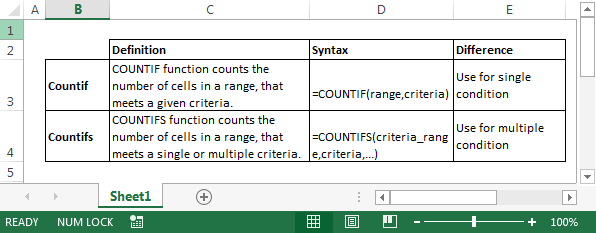Example 1:- Counting number of dates which are greater than then-criteria date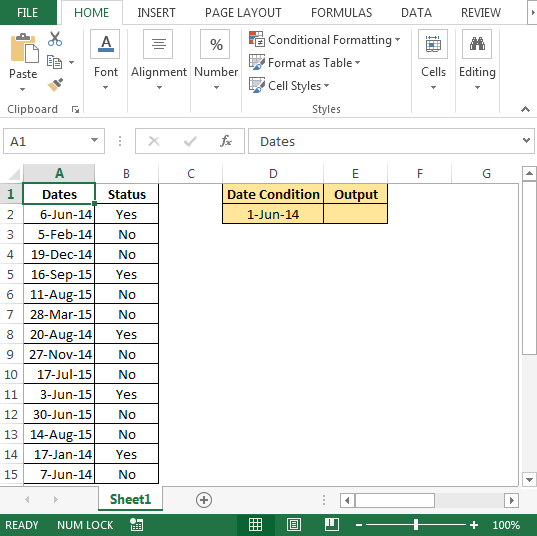• In cell E2, the formula will be
• =COUNTIF(A2:A15,">"&D2)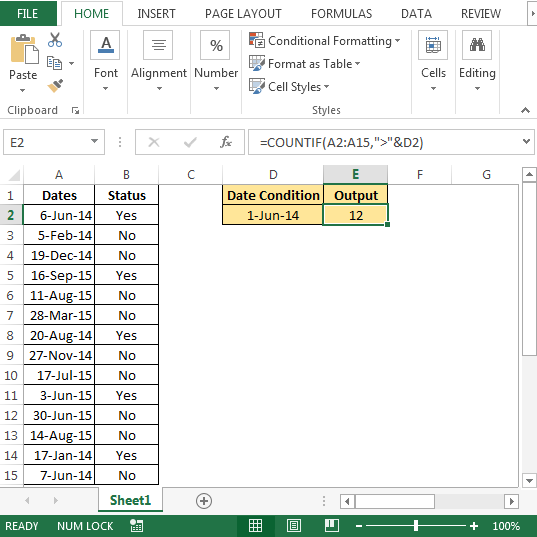Example 2: - Multiple condition: counting number of dates which are greater than then-criteria date with Status “Yes”

• In cell E2 the formula will be
• =COUNTIFS(A2:A15,">"&D2,B2:B15,"Yes")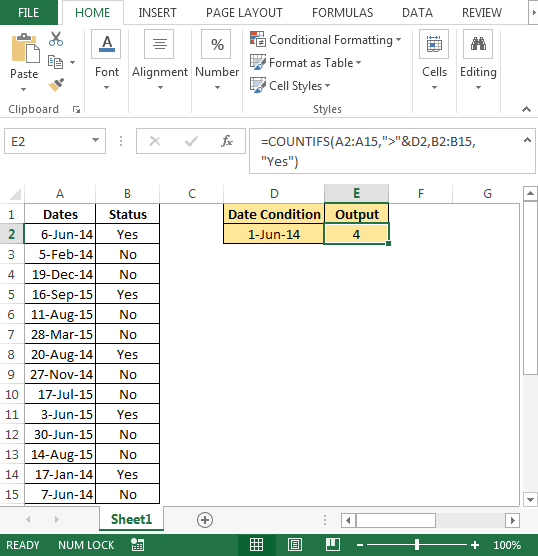Example 3:- COUNTIF & COUNTIFS to get same result (counting number of dates which fall between two criterion dates)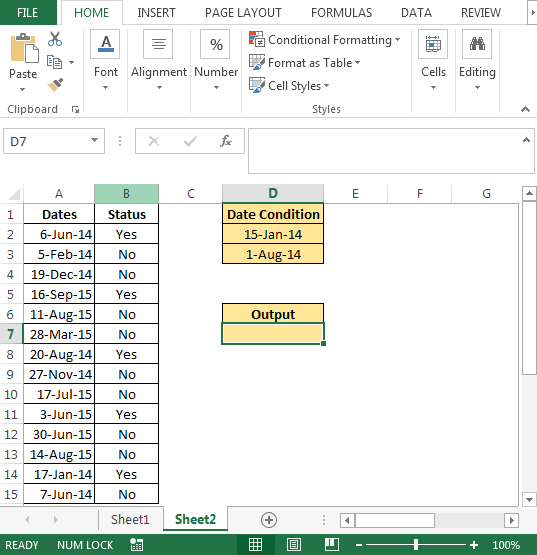• In cell D7, the formula will be
• =COUNTIFS(A2:A15,">"&D2,A2:A15,"<"&D3)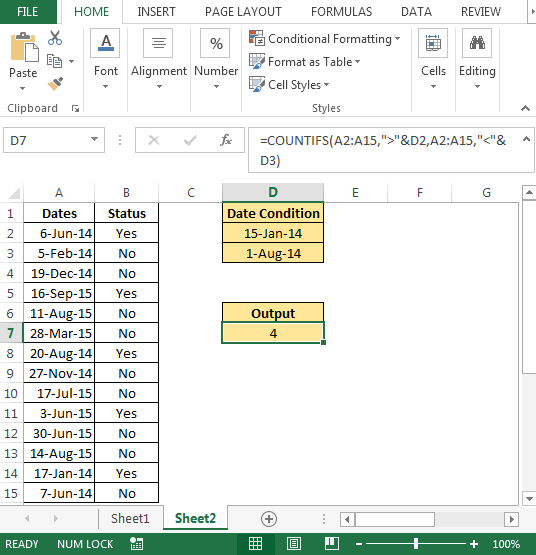The below formula will use only COUNTIF function to get the same result.

• In cell E7, the formula will be
• =COUNTIF(A2:A15,">"&D2)-COUNTIF(\$A2:\$A15,">"&D3)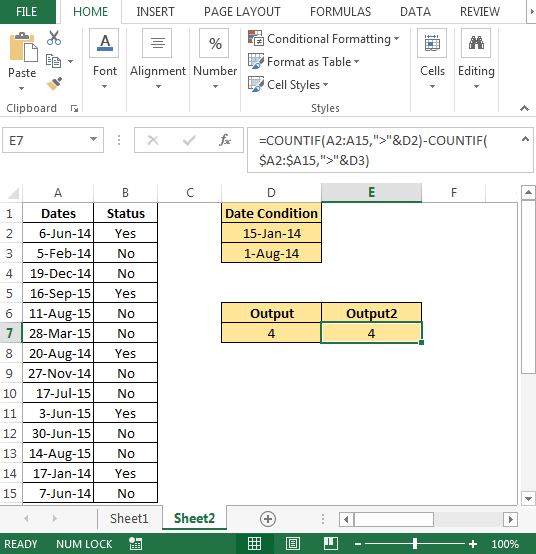Conclusion:- With the help of above examples, we can apply COUNTIF & COUNTIFS function depending on the outcome required to achieve the result.